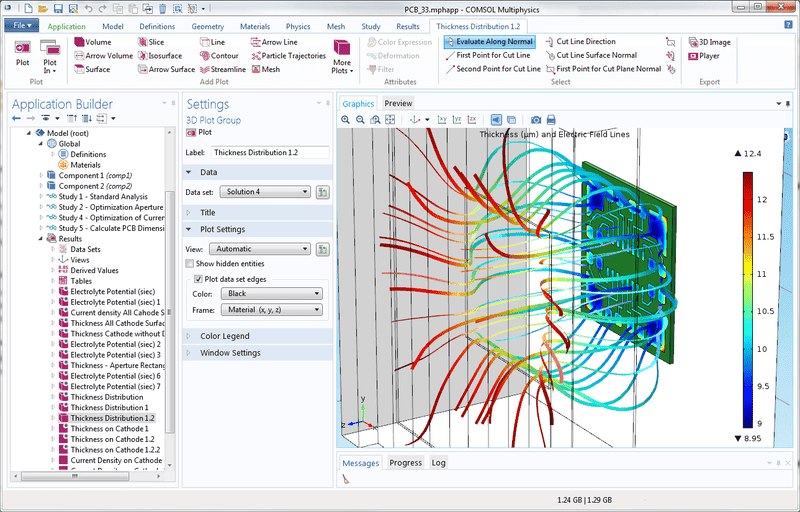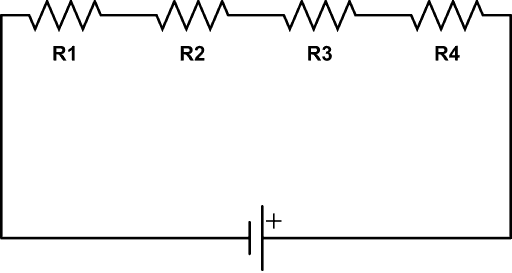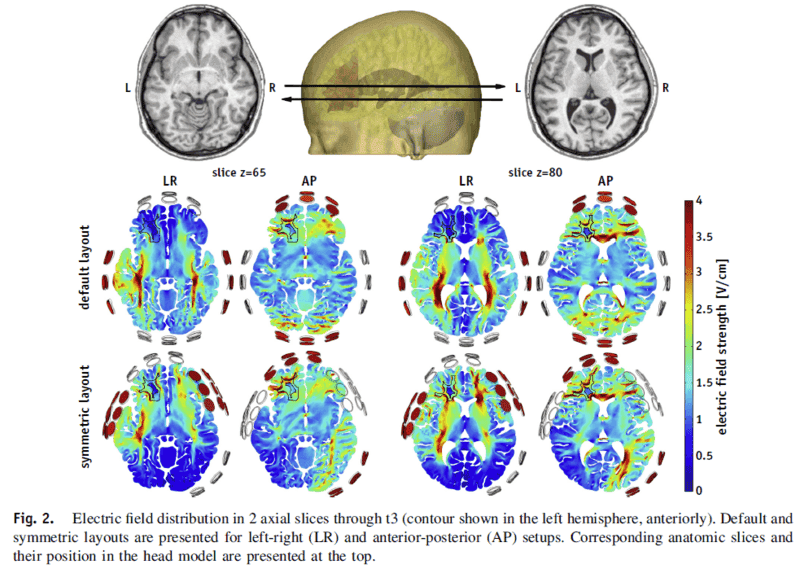# Electric field distribution in the brain

• afmower
In summary, the discussion revolved around the concept of electric field distribution in the brain and how it is affected by factors like distance, tissue properties, and orientation. The question was raised about the relationship between electric field intensity and distribution, and how they are often reported with the same units even though they seem to be different concepts. It was mentioned that the electric field between two infinite plates has the same magnitude and direction at every point in space, while in other situations it can vary. The main focus was on understanding the basic concept of intensity and distribution in a 3D model and the units used to measure them.f

#### afmower

I have a PhD in Molecular Neuroscience but am working on something that requires knowledge of electric field distribution in the brain. I understand that electric field intensity (V/cm) is E=(V1-V2)/d in a theoretical model (between 2 infinite plates, that are parallel and equidistant through a homogeneous medium). In a model where the plates are not parallel and the medium is not homogenous (say the brain for example), field intensity is affected by the distance between the 2 plates, the location and orientation of interfaces between different tissues and the individual dielectric properties of tissues. Here is where I am getting lost...

I often see electric field distribution also given in V/cm. How is this possible when intensity and distribution do not seem to be interchangeable or directly proportional according the following:
-Field distribution in serial tissues of increasing resistance: field distribution across the 3 tissues in series is equal regardless of the resistance and intensity is highest in the tissue of highest resistance
-Filed distribution in parallel tissues with different levels of resistance: field distribution is greatest in the tissue with lowest resistance when tissues are parallel and field intensity is equal regardless of resistance

I have a PhD in Molecular Neuroscience but am working on something that requires knowledge of electric field distribution in the brain. I understand that electric field intensity (V/cm) is E=(V1-V2)/d in a theoretical model (between 2 infinite plates, that are parallel and equidistant through a homogeneous medium). In a model where the plates are not parallel and the medium is not homogenous (say the brain for example), field intensity is affected by the distance between the 2 plates, the location and orientation of interfaces between different tissues and the individual dielectric properties of tissues. Here is where I am getting lost...

I often see electric field distribution also given in V/cm. How is this possible when intensity and distribution do not seem to be interchangeable or directly proportional according the following:
-Field distribution in serial tissues of increasing resistance: field distribution across the 3 tissues in series is equal regardless of the resistance and intensity is highest in the tissue of highest resistance
-Filed distribution in parallel tissues with different levels of resistance: field distribution is greatest in the tissue with lowest resistance when tissues are parallel and field intensity is equal regardless of resistance

Welcome to the PF.I've moved your thread to the Biology/Medical forum for now, since it seems to fit here better.

It would seem that the E-field distributions in the brain would be a combination of the short range fields associated with ion polarization/depolarization during neuron action, combined with aggregate fields that result from summing up the large number of short-range fields. How familiar are you with EEG monitoring?

Welcome to the PF.I've moved your thread to the Biology/Medical forum for now, since it seems to fit here better.

It would seem that the E-field distributions in the brain would be a combination of the short range fields associated with ion polarization/depolarization during neuron action, combined with aggregate fields that result from summing up the large number of short-range fields. How familiar are you with EEG monitoring?
Thank you for your response. I am not familiar with the details of EEG monitoring. Perhaps I should clarify, I am not referring to naturally occurring electric fields in the brain, I am referring to an applied electric field. Perhaps my question would be more straight forward if I made it more general by making the example just be across three medium (not tissues) of increasing resistance. I think I am trying to understand more about the basic concept or relationship between intensity and distribution (particularly in a 3D model) and why I often see them reported with the same units when they don't seem to be interchangeable.

Between two infinite plates, the electric field (which can be thought of as an arrow attached to each point of space) has the same magnitude and points in the same direction at every point in space. In other situations, the electric field has a different magnitude and points in a different direction at different points in space.

Between two infinite plates, the electric field (which can be thought of as an arrow attached to each point of space) has the same magnitude and points in the same direction at every point in space. In other situations, the electric field has a different magnitude and points in a different direction at different points in space.
Yes, I understand that. Is the distribution of an electric field in 3D space measured in V/cm? And thank you for your response by the wayIn my original thread I noted that I am familiar with the concept of electric field intensity (V/cm) is E=(V1-V2)/d in a theoretical model (between 2 infinite plates, that are parallel and equidistant through a homogeneous medium). In a model where the plates are not parallel and the medium is not homogenous (say the brain for example), field intensity is affected by the distance between the 2 plates, the location and orientation of interfaces between different medium and the individual dielectric properties of said medium.

Here is where I am getting lost...

I often see electric field distribution also given in V/cm. How is this possible when intensity and distribution do not seem to be interchangeable or directly proportional according the following:
-Field distribution in serial medium of increasing resistance: field distribution across the 3 medium in series is equal regardless of the resistance and intensity is highest in the medium of highest resistance
-Filed distribution in parallel medium with different levels of resistance: field distribution is greatest in the medium with lowest resistance when they are parallel and field intensity is equal regardless of resistance

Last edited:
Is the distribution of an electric field in 3D space measured in V/cm?
In cgs units, yes. In mks units, it is V/m.
Field distribution in serial medium of increasing resistance: field distribution across the 3 medium in series is equal regardless of the resistance and intensity is highest in the medium of highest resistance
When discussing distributions of E-fields, it's usually the dielectric constant of the medium that is important, not resistivity. If you have active conduction going on, then the resistivity of the materials will matter. But unless the resistivity of the materials is fairly high, the E-fields will be kept low (it doesn't take much of an E-field to drive a current through a good conductor).

When discussing time-varying E-fields, you use the complex impedance of the medium. This can vary with time and/or with position and/or with E-field intensity. Given a complex enough geometry, you would probably model the situation with COMSOL or a similar E&M FEA software package...

https://cdn.comsol.com/products/electrodeposition/pcb_results_mph.png#### Attachments

•jim mcnamara
Also, I should add that the E-field and other quantities are generally vectors, so all of the math you would use to make these calculations will be vector-based.

Last edited:
Also, I should add that the E-field and other quantities like dielectric constant, conductivity, etc. are vectors, so all of the math you would use to make these calculations will be vector-based.
Thank you for your response. If the impact of resistance or in this case, dielectric properties, is different on distribution versus intensity, which I am assuming based on the simplified example below, what is the correlation between the 2 in a heat map such as that pictured in your example. There is not a direct relationship between more concentrated or dense distribution and intensity, correct? In other words, where intensity is highest on the heat map, that does not necessarily mean that distribution is highest/most concentrated, correct?

Example:
-Field distribution in serial medium of increasing resistance: field distribution across the 3 medium in series is equal regardless of the resistance and intensity is highest in the medium of highest resistance
-Filed distribution in parallel medium with different levels of resistance: field distribution is greatest in the medium with lowest resistance when they are parallel and field intensity is equal regardless of resistance

I'm not really understanding what you are asking, but I'll try this approach...
-Field distribution in serial medium of increasing resistance: field distribution across the 3 medium in series is equal regardless of the resistance and intensity is highest in the medium of highest resistance
Can you model this by using simple resistors and Ohm's Law for series resistors?-Filed distribution in parallel medium with different levels of resistance: field distribution is greatest in the medium with lowest resistance when they are parallel and field intensity is equal regardless of resistance
And model this with parallel resistors, which all have the same voltage across them, and their current is determined by their individual resistances I = V/R

https://www.electronics-tutorials.ws/resistor/res50.gif

https://www.electronics-tutorials.ws/resistor/res50.gif

#### Attachments

I'm not really understanding what you are asking, but I'll try this approach...

Can you model this by using simple resistors and Ohm's Law for series resistors?

View attachment 229446

And model this with parallel resistors, which all have the same voltage across them, and their current is determined by their individual resistances I = V/R

https://www.electronics-tutorials.ws/resistor/res50.gif

https://www.electronics-tutorials.ws/resistor/res50.gif
Thanks again. Perhaps I am just getting confused by semantics. LOLI'm not really understanding what you are asking, but I'll try this approach...

Can you model this by using simple resistors and Ohm's Law for series resistors?

View attachment 229446

And model this with parallel resistors, which all have the same voltage across them, and their current is determined by their individual resistances I = V/R

https://www.electronics-tutorials.ws/resistor/res50.gif

https://www.electronics-tutorials.ws/resistor/res50.gif
Maybe this is a really stupid question but what is the difference between distribution and intensity since they are measured in the same units?

Maybe this is a really stupid question but what is the difference between distribution and intensity since they are measured in the same units?
I would think by "distribution", we would be referring to the shape of the field lines. By "intensity", we would mean the magnitude of the E-field at a point. Can you link to an article that uses both of those terms?

I would think by "distribution", we would be referring to the shape of the field lines. By "intensity", we would mean the magnitude of the E-field at a point. Can you link to an article that uses both of those terms?
Thank you for your continued insights. Take the image below for example (t3-tumor #3). It seems that field distribution and field strength are being used interchangeably. Is it just that the figure legend should technically say electric field intensity distribution in (V/cm)? Hence what I meant by getting confused by semantics. LOL#### Attachments

Just to help narrow down the context, is this in regard to tomography or to localized stimulation by external fields?

If I understand what you're asking, the term "distribution" usually means a map of intensity values. The electric field has a magnitude (and direction) for every point in space. They have plotted out the magnitude of the electric field for all points in each of the axial images of the brain. This magnitude is the "strength" at any given point. Pixels that are dark red correspond to a field strength of 4 V/cm. For those that are dark blue, the value is 0 V/cm. So the magnitude of the electric field (or "strength" or "intensity") is different at any given point. Collectively the "map" of all of these different values is the "distribution."

•berkeman and jim mcnamara
The OPs question is in regard to an externally applied voltage to the Human brain as a treatment for glioblastoma (Brain Cancer). The image the OP supplied is from the research report here.

It is a COMSOL simulation of an array of small electrodes applied to the scalp and a 200kHz voltage is applied to selected electrodes. A field strength above 1V/cm disrupts the growth of tumor cells. The report states that there is an increase in field strength at tumor margins, but to me that was not obvious.

I think @Choppy got the 'field strength' vs 'distribution' explanation.
the magnitude of the electric field (or "strength" or "intensity") is different at any given point. Collectively the "map" of all of these different values is the "distribution."

•berkeman
Don't know how much this helps, however, the cortex might act as a low pass filter when transmitting to deep brain structures.

Last edited: